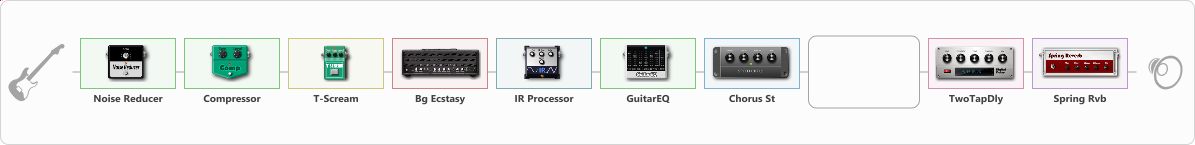Discussion in 'ToneLib-GFX presets' started by Evgeniy ESP, Aug 12, 2022.

Preset name: bogner

Effects chain:Effect: "Noise Reducer" (Dynamics / Filter), active - "yes"
{
"Sens" = 81
"Mode" = Soft
}

Effect: "Compressor" (Dynamics / Filter), active - "yes"
{
"Sense" = 21
"Level" = 42
}

Effect: "T-Scream" (Overdrive / Distortion), active - "yes"
{
"Drive" = 20
"Tone" = 80
"Level" = 84
}

Effect: "Bg Ecstasy" (Amp simulators), active - "yes"
{
"Gain" = 46
"Bass" = 62
"Middle" = 50
"Treble" = 66
"Presence" = 66
"Master" = 50
"Level (dB)" = 0
}

Effect: "IR Processor" (Cabinets), active - "yes"
{
"IR" = s-pres5
"Low Cut (Hz)" = 81
"Hi Cut (kHz)" = 15.0
"Mix" = 100
"Level (dB)" = 0
}

Effect: "GuitarEQ" (Dynamics / Filter), active - "yes"
{
"160 Hz" = 0
"400 Hz" = -1
"800 Hz" = -1
"1.6 kHz" = -1
"3.2 kHz" = 0
"6.4 kHz" = 0
"12 kHz" = -1
"Level (dB)" = 0
}

Effect: "Chorus St" (Modulation / Sfx), active - "yes"
{
"Speed" = 0.1
"Depth" = 0
"Center" = 4.4
"Mix" = 73
}

Effect: "Splitter" (Dynamics / Filter)
{
"A-Bypass" = Off
"A-Pan" = -25
"A-Level" = 59
"B-Bypass" = Off
"B-Pan" = 25
"B-Level" = 59
"Width" = 52

'A' branch:
{
}
'B' branch:
{
}
}

Effect: "TwoTapDly" (Delay), active - "yes"
{
"Time" = 756
"Feedback" = 44
"Tone" = 60
"Sens" = 0
"TapTime" = 5.0
"Mix" = 56
}

Effect: "Spring Rvb" (Reverberation), active - "yes"
{
"Time" = 10.0
"PreDelay" = 0
"LoDamp" = 16
"HiDamp" = 26
"Mix" = 42
}

Note: You will need to download and install the ToneLib-GFX software to use the preset.